Worksheets and No Prep Teaching Resources Math Third Grade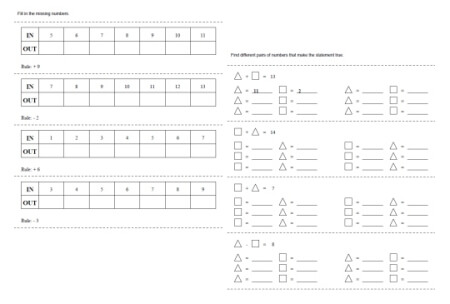Third Grade Algebra Printables, Worksheets, and Lessons

Third Grade Algebra Mixed Review Book

Change in Numbers: How Did it Change?
Numbers 1-9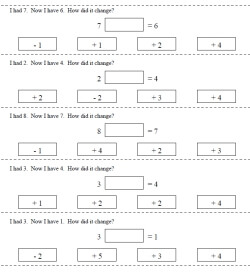Numbers 1 to 9 - the change will be from 1 to 3 - equation is given Numbers 1 to 9 - the change will be from 1 to 5 - equation is given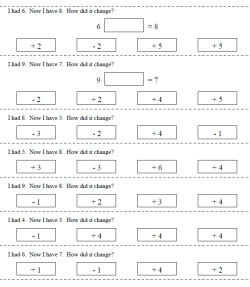Numbers 1 to 9 - the change will be from 1 to 3 - equation is given in HALF of the problems Numbers 1 to 9 - the change will be from 1 to 5 - equation is in HALF of the problems given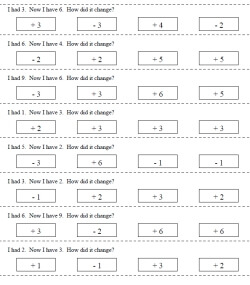Numbers 1 to 9 - the change will be from 1 to 3 - no equation help Numbers 1 to 9 - the change will be from 1 to 5 - no equation help

Numbers 1-19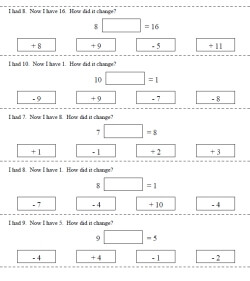Numbers 1 to 9 - the change will be from 1 to 9 - equation is given Numbers 1 to 9 - the change will be from 1 to 18 - equation is given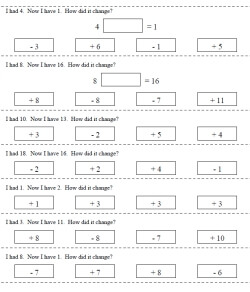Numbers 1 to 9 - the change will be from 1 to 9 - equation is given in HALF of the problems Numbers 1 to 9 - the change will be from 1 to 18 - equation is in HALF of the problems given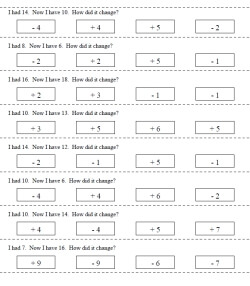Numbers 1 to 9 - the change will be from 1 to 9 - no equation help Numbers 1 to 9 - the change will be from 1 to 18 - no equation help

Patterns
Circle Growing or Decreasing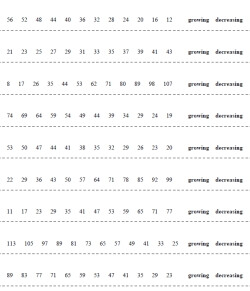Circle growing or decreasing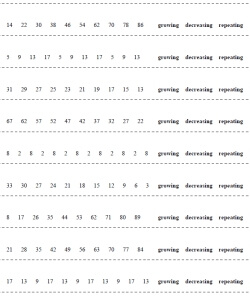Circle growing, decreasing, or repeating

Complete the Sequence - Change Boxes Given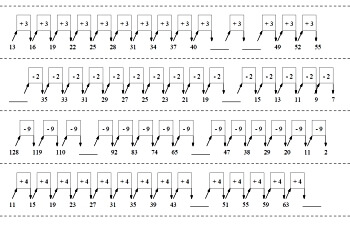Growing or Decreasing: Complete the pattern - all the change values are given - 2 blanks Growing or Decreasing: Complete the pattern - all the change values are given - 4 to 6 blanksGrowing, Decreasing, or Repeating: Complete the pattern - all the change values are given - 2 blanks Growing, Decreasing, or Repeating: Complete the pattern - all the change values are given - 4 to 6 blanks

Write the Rule - Change Boxes Given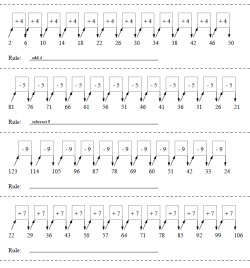Write the Rule: Growing or Decreasing (rule given for the first two problems) Write the Rule: Growing or Decreasing

Write the Rule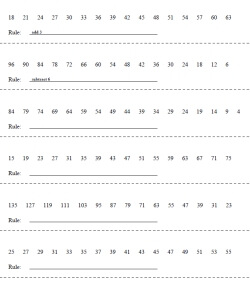Write the Rule: Growing or Decreasing (rule given for the first two problems)

Complete the Sequence and Fill-In Change Boxes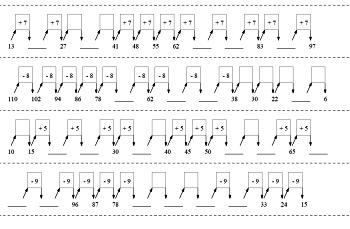Complete the pattern - 2 change values are blank - 2 blanks Complete the pattern - 2 to 4 change values are blank - 4 to 6 blanks

Complete the Sequence - Repeating Numbers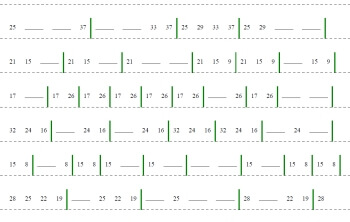Repeating: Complete the pattern - green lines to show repeating (2 to 4 blanks; landscape page) Repeating: Complete the pattern - green lines to show repeating (4 to 6 blanks; landscape page)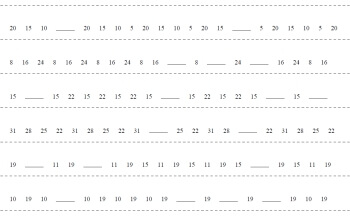Repeating: Complete the pattern (2 to 4 blanks; landscape page)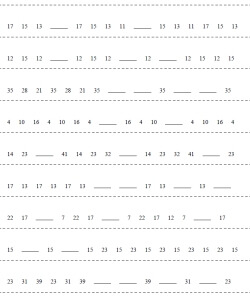Repeating: Complete the pattern (2 to 4 blanks) Challenging - Repeating: Complete the pattern (4 to 6 blanks)

Complete the Sequence - Growing or Decreasing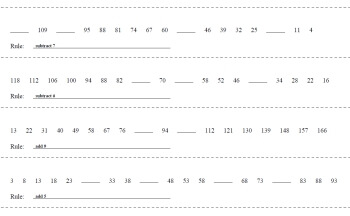Growing or Decreasing: Complete the pattern - Rule is Given (2 to 4 blanks; landscape page) Growing or Decreasing: Complete the pattern - Rule is Given (4 to 6 blanks; landscape page)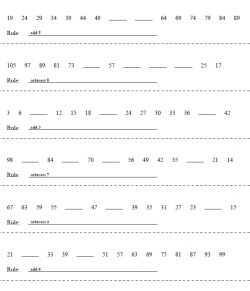Growing or Decreasing: Complete the pattern - Rule is Given (2 to 4 blanks) Growing or Decreasing: Complete the pattern - Rule is Given (4 to 6 blanks)

More Patterns

Patterns and Functions: IN and OUT
Easier Functions: The Rule is Given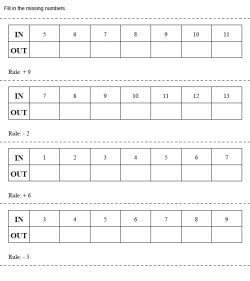Easy: Rule is given, IN are numbers that increase by 1, Fill in OUT, addition or subtraction, horizontal display Easy: Rule is given, IN are numbers that increase by 1, Some IN missing and some OUT, addition or subtraction, horizontal display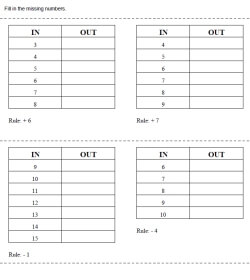Easy: Rule is given, IN are numbers that increase by 1, Fill in OUT, addition or subtraction, vertical display Easy: Rule is given, IN are numbers that increase by 1, Some IN missing and some OUT, addition or subtraction, vertical display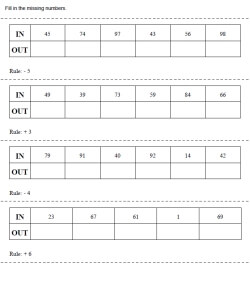Easy: Rule is given, IN values are random, Fill in OUT, addition or subtraction, horizontal display Easy: Rule is given, IN values are random, Some IN missing and some OUT, addition or subtraction, horizontal display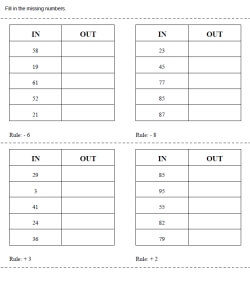Easy: Rule is given, IN values are random, Fill in OUT, addition or subtraction, vertical display Easy: Rule is given, IN values are random, Some IN missing and some OUT, addition or subtraction, vertical display

Rules Listed on Top of Page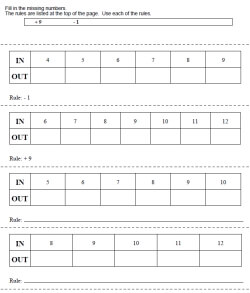Easy: Rule is given, IN are numbers that increase by 1, Fill in OUT, addition or subtraction, horizontal display Easy: Rule is given, IN are numbers that increase by 1, Some IN missing and some OUT, addition or subtraction, horizontal display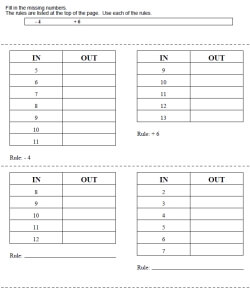Easy: Rule is given, IN are numbers that increase by 1, Fill in OUT, addition or subtraction, vertical display Easy: Rule is given, IN are numbers that increase by 1, Some IN missing and some OUT, addition or subtraction, vertical display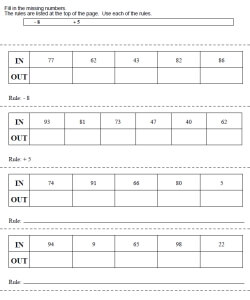Easy: Rule is given, IN values are random, Fill in OUT, addition or subtraction, horizontal display Easy: Rule is given, IN values are random, Some IN missing and some OUT, addition or subtraction, horizontal display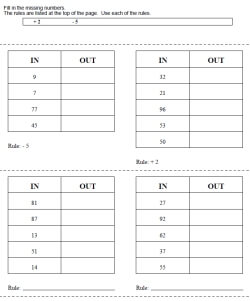Easy: Rule is given, IN values are random, Fill in OUT, addition or subtraction, vertical display Easy: Rule is given, IN values are random, Some IN missing and some OUT, addition or subtraction, vertical display

Patterns and Functions: IN and OUT with Shapes
Easier Functions: The Rule is Given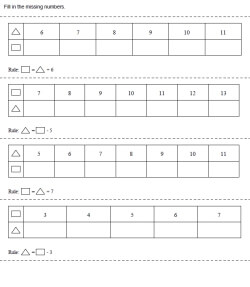Easy: Rule is given, IN are numbers that increase by 1, Fill in OUT, addition or subtraction, horizontal display Easy: Rule is given, IN are numbers that increase by 1, Some IN missing and some OUT, addition or subtraction, horizontal display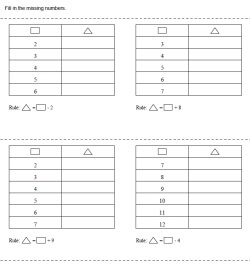Easy: Rule is given, IN are numbers that increase by 1, Fill in OUT, addition or subtraction, vertical display Easy: Rule is given, IN are numbers that increase by 1, Some IN missing and some OUT, addition or subtraction, vertical display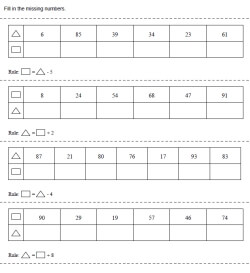Easy: Rule is given, IN values are random, Fill in OUT, addition or subtraction, horizontal display Easy: Rule is given, IN values are random, Some IN missing and some OUT, addition or subtraction, horizontal display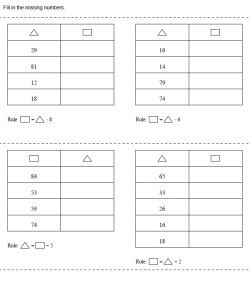Easy: Rule is given, IN values are random, Fill in OUT, addition or subtraction, vertical display Easy: Rule is given, IN values are random, Some IN missing and some OUT, addition or subtraction, vertical display

Sequences

Possible Number Solutions - Greater and Less Than - Number Sentences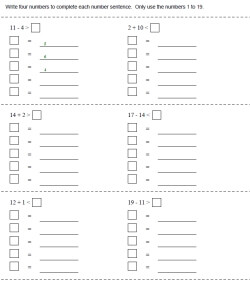Numbers to 9 (some answers given in first problem) Numbers to 19 (some answers given in first problem) Numbers to 49 (some answers given in first problem)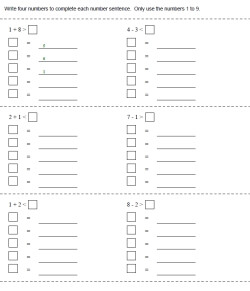Numbers to 9 Numbers to 19 Numbers to 49

Write the Equation - Unscramble to Make an Equation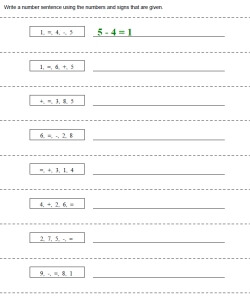Numbers to 9: Addition Only (first answer given) Numbers to 9: Subtraction Only (first answer given) Numbers to 9: Addition or Subtraction (first answer given)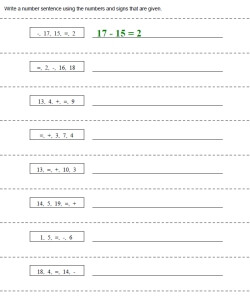Numbers to 19: Addition Only (first answer given) Numbers to 19: Subtraction Only (first answer given) Numbers to 19: Addition or Subtraction (first answer given)

Making a Number Sentence: Unknowns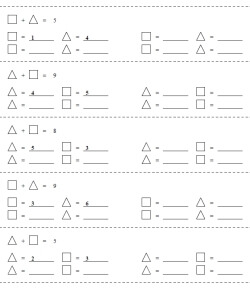Maximum sum of 9 - one answer given for each problem Maximum sum of 19 - one answer given for each problem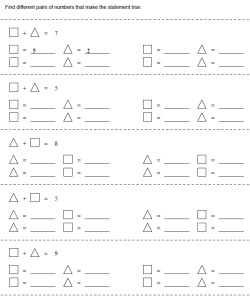Maximum sum of 9 - one answer given for first two problem Maximum sum of 9 - one answer given for first two problem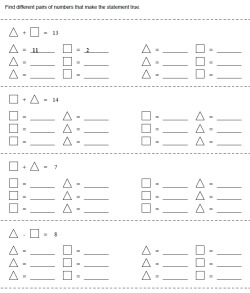Maximum sum of 9 - one answer given for first two problem - give six solutions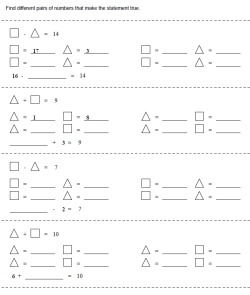Maximum sum of 9 - one answer given for first two problem - one equation where two numbers are given

Ordered Pairs

Greater and Less Than: Make the Scale Balance
Easier: Maximum Sum of 19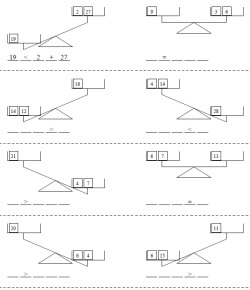Answer given for the first problem - the sign is given for all the problems Answer given for the first problem - the sign is given for the first four problems Answer given for the first problem No answers, but blank lines are shown for parts ___ ____ ____ ____ _____
Maximum Sum of 99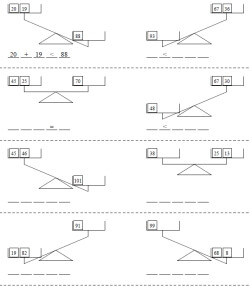Answer given for the first problem - the sign is given for all the problems Answer given for the first problem - the sign is given for the first four problems Answer given for the first problem No answers, but blank lines are shown for parts ___ ____ ____ ____ _____
Algebra Challenge - Maximum Sum of 19 - All balances are equal and there is one UNKNOWN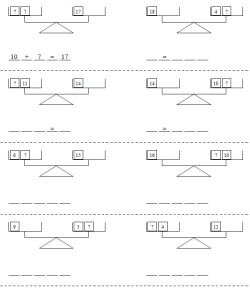Algebra Challenge: Answer given for the first problem - the sign is given for all the problems Algebra Challenge: Answer given for the first problem - the sign is given for the first four problems Algebra Challenge: Answer given for the first problem Algebra Challenge: No answers, but blank lines are shown for parts ___ ____ ____ ____ _____

Have a suggestion or would like to leave feedback?